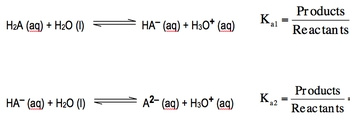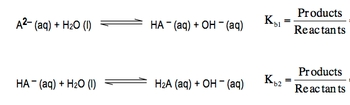## General Chemistry

Learn the toughest concepts covered in Chemistry with step-by-step video tutorials and practice problems by world-class tutors

17. Acid and Base Equilibrium

# Diprotic Acid

A diprotic acid is an acid that can donate two hydronium ions (H+).

Diprotic Acids

Since a diprotic acid has two acidic hydrogens it would have 2 equilibrium equations.

1
concept

## Diprotic Acids3m
Play a video:
in this video, we'll take a look at die product acids. Now we're gonna stay here that die product acids and bases are compounds that can donate or accept to H plus ions. Now let's talk about die product acids. First, we're gonna say Die product acids. The general formula for them will be H two A. That's if you don't know what the dye product as it looks like. But you know what? The dye product as it so it has to acidic hydrogen. That's why it's H two and then a represents the nominal portion. Okay, now they're equations can be illustrated by when we're dealing with di product acids. That means we can donate H plus two times. The first H plus that we donate is associated with K one. Okay, so that's your acid association, Constant one. Then, if you're gonna give away the second acidic hydrogen that deals with K two, what you need to realize here about K one and K two is that K one will always be greater than K two. It's always easier to donate the first H plus and then much more difficult to donate the second hydrogen and in fact, they're usually different by several magnitudes. What I mean by that is a k A one. Let's say it Waas 1.3 times 10 to the negative too. You would expect your K two to be much smaller than that. Maybe like 8.5 times 10 to the negative five. I'm just making up these numbers, but I'm illustrating how they're different by several magnitudes here. This is to the negative to whereas this is to the negative five. So they're different by it. Magnitude of three here. Okay, so there's a big difference in strength. Now, if we're looking at our equilibrium equation, remember H two way this will represent our acid water. He will represent our base. Remember? Based on the Bronston Laurie idea of acids and bases, acids donate H plus bases except H plus. So now, my ass, it has become my conjugate base and water accepted in H plus. So now it's H +30 plus. So this is the conjugate acid. If we're talking about the equilibrium expression that means K one equals products overreact. INTs remember, we ignore solids and liquids. Water is a liquid, so it'll be ignored. So this would be a check a minus times H plus divided by H two Way. Now, after we've given away the first acidic hydrogen we have. This left this because there's still another H plus in it. It has the potential to donate that second one if it decides to donate that second H plus. Then we come down here to this equation. So here it is now it's already given away the first H plus. Now it's about to give away the second one. So again it's the acid water is gonna act as the base, so it's gonna give away an H plus. As a result, it becomes even more negative. So now it's a two minus. So this would be the conjugate base here. Water accepted an H plus. So now it's the conjugate acid we're talking about giving away its second acidic hydrogen. So now we're dealing with K two, and so this would be a to minus times H 30 plus, divided by H A minus. So these will be our to equilibrium expressions dealing with di protic acid, donating it's H plus ions, so give yourself a minute or two to absorb the information that we've just presented in terms of die product acids and then we'll move on to die product basis.The diprotic bases would also have 2 equilibrium equations.

2
concept

## Diprotic Acids2m
Play a video:
now looking at die product basis, We're gonna say the general formula is a to minus because it has a to minus. There, it can accept to h plus is one at a time. Now for those equations, let's take a look. So here we're dealing with the first part of a die product base. It's a to minus form, so it's gonna act as the base so water will be the acid again. Remember, acids donate H plus so that a to minus, except on a H plus to become H A minus water, as a result, becomes O. H minus here. Since we're accepting the first H Plus, we're dealing with K B one are based association constant and just like a one in K two K B one will always be greater than K B two, meaning that it's always easiest to accept that first H plus than it is to accept that second H plus. So here it be H A minus times O. H minus, divided by a two minus. Then, finally, we're gonna say here that we have the possibility of this accepting the second H plus, So if it does, it gives us the second equation here. So again, water is gonna act as the acid. This will be my base. We're gonna donate an H plus. So now it becomes ht way, which is the conjugate acid, all h minus of the conjugate base. So here, up here, this could also be the conjugate acid. And this is the conjugate base again, we're accepting the H plus here for a second time. So we're dealing with baby, too. We ignore solids and liquids. So this is Htwe times of H minus, divided by H A minus. So those were the equilibrium expressions for whether you're dealing with the dye product acid form of the die basic form. Knowing those are key to helping you set up your equilibrium expressions and from there determining your P h and P o h. Now that we've seen, this will continue onward to the last remaining portions when dealing with di product acids and bases3
concept

## Diprotic Acids4m
Play a video:
So based on what we've seen so far, we're gonna say, based on these equations, the relationship between the different forms off died, product species are all right. So before it's given away any of its H plus values, it's going to be Htwe. So you could think of this as it's acidic form. When he gives away the first H plus, it has become H A minus. We'll call this the intermediate form and then we have it giving away its second H plus to become a to minus. So it's lost. All of its H plus is now, so this would be its basic form. These would be the three major forms of any dye product acid or base. Now, as a result of having multiple K s and KBS, we can't just simply say anymore that K Times K B equals K w. Now you have to remember that K one is connected to K B two and multiplying them together gives us our K W, which is still 1.0 times 10 to the negative 14 when the temperature is 25 degrees Celsius and then remember that a k A to is connected to k B one and they're both still connected to K. W. Now, why is that? Well, if we take a look here at the three forms, we're gonna say we're going from the full it fully acid acid form. And we're giving away our first H plus to give us our intermediate form. So the relationship between them is connected by K one. Then if we're giving away the second acidic hydrogen, that's gonna give us K two, and that's what we're looking at it this way. Now, if we look at it the opposite way going this way, this is its basic form in the very beginning, and it accepts in H Plus to become this form. If we're talking about it accepting its first H plus, we're dealing with CBI one. So notice here that K B one and K two are connected here. Then if we're talking about accepting our second H plus to give us back our acidic form, that means we're talking about CBI, too. So that's why K one and K B two are connected, and that's why K two and K B one are connected. So just remember the relationships between those acid Constance and based Constance. Now we're gonna stay here when dealing with di product acids. What? We're trying to figure out our pH. We're gonna say H two way can be treated as the mono product acid in the same way and in that case would use K one. Then, for the intermediate form, we would use K two because it give away its second acidic hydrogen to become just like the base. Then we'd say that a to minus represents the basic foreman in that case would use K B one and technically, for the intermediate form, we could use either que two or k B two. Depending on. Are we is this thing accepting an H plus? If it's accepting an H plus, then we'd use K B two. Is it giving away an H plus? In that case, we use K two, so the intermediate forms a little bit tricky. It's all based on. Is it acting as an acid, or is it acting as a base? Because, remember, here it has ah, hydrogen in front and a negative charge in the back so it can act as either an acid or base because it is anfo Terek. And because of that, we could go and use K two if it's acting, hacking acid and donating an H plus. Or we could use K B two if it's gonna accept an H plus and act like a base. So these are just some of the fundamental principles you need to keep in mind when dealing with di product acids and bases. As we go further into this concept will look at problems and we'll learn. Learn how to approach those different types of questions to figure out what our pH or P O. H will be.
4
example

## Diprotic Acids13m
Play a video: